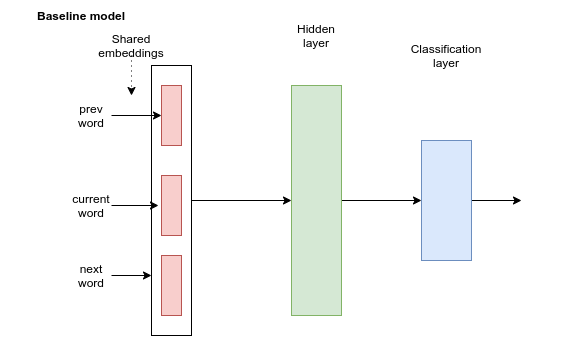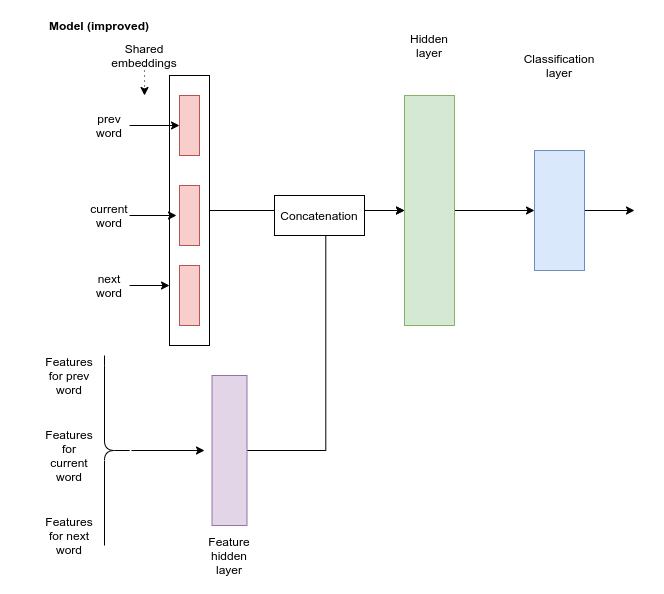top of page
Search

# Implementing NLP Models For Name Entity Recognition | Hire NLP Expert

Requirement Details

We will incorporate word features (suffixes and prefixes) into the model, in addition to the word embeddings. Your task is to implement two models that use the features in slightly different ways.Import Necessary Packages

```import numpy as np
import torch

```!wget -q https://raw.githubusercontent.com/synalp/NER/master/corpus/CoNLL-2003/eng.train

!wget -q https://raw.githubusercontent.com/synalp/NER/master/corpus/CoNLL-2003/eng.testa```

```# Convert the CONLL data to our format
from itertools import chain, groupby
result = []
f = open(filename)
lines = (str.strip(line) for line in  f)
groups = (grp for nonempty, grp in groupby(lines, bool) if nonempty)

for group in groups:
group = list(group)

obs, lbl = zip(*(ln.rsplit(None, 1) for ln in group))
lbl = [l.lstrip("B-").lstrip("I-") for l in lbl]
word = [x.split() for x in obs]

result.append(list(zip(word, lbl)))
return result```

```train_data = read_conll("eng.train")
```from collections import Counter
word_counter = Counter()
for sentence in train_data:
for word, label in sentence:
word_counter[word] += 1```
```vocabulary = {}
vocabulary["<unk>"] = 0
vocabulary["<s>"] = 1
vocabulary["</s>"] = 2
for word in word_counter:
if word_counter[word] > 1:
vocabulary[word] = len(vocabulary)

label_vocabulary = {}
label_vocabulary["O"] = 0
label_vocabulary["ORG"] = 1
label_vocabulary["LOC"] = 2
label_vocabulary["MISC"] = 3
label_vocabulary["PER"] = 4```

The above was exactly as we did the lab. Now, we also extract the word features.

First, we implement a function that generates 'candidate' features for each word. We use prefixes and suffixes of length 2 and 3.

We use the cached decorator to cache the features for frequent words.

```from cachetools import cached, LRUCache, TTLCache
@cached(cache={})
def generate_word_feature_candidates(word):
result = set()
for i in [2, 3]:
return result```
`print(generate_word_feature_candidates("Brexit"))`

Next, we count the feature occurrences in training data:

```feature_counter = Counter()

for sentence in train_data:
for word, label in sentence:
features = generate_word_feature_candidates(word)
for feature in features:
feature_counter[feature] += 1```

Next, we make a feature-to-int mapping for the features that occur at least 50 times

```feature_vocabulary = {}
feature_list = []

feature_threshold =  50
for feature in feature_counter:
if feature_counter[feature] >= feature_threshold:
feature_vocabulary[feature] = len(feature_vocabulary)
feature_list.append(feature)```

Some info about the kept features:

```print(len(feature_vocabulary))
print(list(feature_vocabulary.items())[0:20])
print(feature_list[0:20])```

output:

1833 [('__T-', 0), ('-DO__', 1), ('-D__', 2), ('__RT-', 3), ('__ts', 4), ('__cts', 5), ('re__', 6), ('Ger__', 7), ('Ge__', 8), ('__man', 9), ('__an', 10), ('__all', 11), ('__ll', 12), ('ca__', 13), ('cal__', 14), ('__to', 15), ('to__', 16), ('bo__', 17), ('__tt', 18), ('Bri__', 19)] ['__T-', '-DO__', '-D__', '__RT-', '__ts', '__cts', 're__', 'Ger__', 'Ge__', '__man', '__an', '__all', '__ll', 'ca__', 'cal__', '__to', 'to__', 'bo__', '__tt', 'Bri__']

The next function find the feature IDs for a word. It first generates feature candidates for a word, and then keeps only those that are in our feature vocabulary:

```@cached(cache={})
def get_word_feature_ids(word):
feature_candidates = generate_word_feature_candidates(word)
result = []
for feature in feature_candidates:
feature_id = feature_vocabulary.get(feature, -1)
if feature_id >= 0:
result.append(feature_id)
return result```

There feature IDs for the word 'Brexit':

`get_word_feature_ids("Brexit")`

Feature IDs and feature string reprsenatations wor the word 'Tallinn':

`print([(i, feature_list[i]) for i in get_word_feature_ids("Tallinn")])`

output:

[(638, 'Ta__'), (125, '__nn')]

Now, we include the feature extraction part in our dataset. Note that now, each data item (word observation) will consists of 3 items: words (left word, current word, right word), features for the left, current and right word, and the label (y):

```class NERDataset(Dataset):
"""Name Classification dataset"""

def __init__(self, data ):
words = []
self.features = []
labels = []
for sentence in data:
for i in range(len(sentence)):
if i > 0:
prevw = vocabulary.get(sentence[i-1], 0)
prevw_features = get_word_feature_ids(sentence[i-1])
else:
prevw = vocabulary["<s>"]
prevw_features = []
if i+1 < len(sentence):
nextw = vocabulary.get(sentence[i+1], 0)
nextw_features = get_word_feature_ids(sentence[i+1])
else:
nextw = vocabulary["</s>"]
nextw_features = []
words.append((prevw, vocabulary.get(sentence[i], 0), nextw))
self.features.append((prevw_features,  get_word_feature_ids(sentence[i]), nextw_features))

labels.append(label_vocabulary[sentence[i]])
self.words = torch.from_numpy(np.array(words).astype(int)).long()

self.y = torch.from_numpy(np.array(labels).astype(int)).long()

def __len__(self):
return len(self.words)

def __getitem__(self, index):
words = self.words[index]
feature_matrix = torch.zeros(3, len(feature_vocabulary), dtype=torch.uint8)
for j in range(3):
for k in self.features[index][j]:
feature_matrix[j, k] = 1

y = self.y[index]

sample = {'words': words, 'features': feature_matrix, 'y': y}
return sample```
```train_dataset = NERDataset(train_data)
dev_dataset = NERDataset(dev_data)```

Let's check how our first data item looks like. Note that 'features' is a 3x1833 tensor -- the rows corresponds to previous, current and next word and columns correspond to features. The tensor consists of mostly zeros, only in places where a certain feature is activated there is a one.

`train_dataset`

output:

{'features': tensor([[0, 0, 0, ..., 0, 0, 0], [1, 1, 1, ..., 0, 0, 0], [0, 0, 0, ..., 0, 0, 0]], dtype=torch.uint8), 'words': tensor([1, 3, 2]), 'y': tensor(0)}

Next part implements our baseline model that only uses word embeddings. Note that the forward method actually takes feature tensor as an argument, but it is not used in this model.

```import torch.nn as nn
import torch.nn.functional as F

class NERNN(nn.Module):

def __init__(self, vocab_size, embedding_dim, hidden_dim, output_size):
super(NERNN, self).__init__()
self.fc1 = nn.Linear(embedding_dim * 3, hidden_dim)
self.fc2 = nn.Linear(hidden_dim, output_size)

def forward(self, words, features):
x = self.embeddings(words).view(-1, (embedding_dim * 3))
x = F.relu(self.fc1(x))
x = self.fc2(x)
y = F.log_softmax(x, dim=1)
return y```
```device = 'cpu'
if torch.cuda.is_available():
device = torch.device('cuda')
print(device)

```

The next part implements training and evaluation. It has also been enhanced so that it feeds the feature tensor to the model.

```def train(model, num_epochs, train_iter, test_iter):

steps = 0
best_acc = 0
last_step = 0
for epoch in range(1, num_epochs+1):
print("Epoch %d" % epoch)
model.train()
for batch in train_iter:
words, features, y = batch['words'].to(device), batch['features'].to(device), batch['y'].to(device)

output = model(words, features)

loss = F.nll_loss(output, y)
loss.backward()
optimizer.step()

steps += 1

print('  Epoch finished, evaluating...')
train_acc = evaluate("train", train_iter, model)
dev_acc = evaluate("test", test_iter, model)

def evaluate(dataset_name, data_iter, model):

model.eval()
total_corrects, avg_loss = 0, 0
# the following disables gradient computation for evaluation, as we don't need it
for batch in data_iter:
words, features, y = batch['words'].to(device), batch['features'].to(device), batch['y'].to(device)

output = model(words, features)

loss = F.nll_loss(output, y, reduction='sum').item() # sum up batch loss
pred = output.argmax(dim=1, keepdim=True) # get the index of the max log-probability
correct = pred.eq(y.view_as(pred)).sum().item()

avg_loss += loss

total_corrects += correct

size = len(data_iter.dataset)
avg_loss /= size
accuracy = 100.0 * total_corrects/size
print('  Evaluation on {} - loss: {:.6f}  acc: {:.4f}%({}/{})'.format(dataset_name,
avg_loss,
accuracy,
total_corrects,
size))
return accuracy                ```

Let's define some constants that we need for he model:

```vocab_size = len(vocabulary)
embedding_dim = 50 # dimensionality of word embeddings
hidden_dim = 100   # dim of hidden layer
output_size = len(label_vocabulary) # number of classes```

Now, let's train the baseline model:

```train_iter = DataLoader(train_dataset, batch_size=64, shuffle=True)
model = NERNN(vocab_size, embedding_dim, hidden_dim, output_size).to(device)
train(model, 5, train_iter, dev_iter)```

output:

Epoch 1 Epoch finished, evaluating... Evaluation on train - loss: 0.232839 acc: 92.4494%(189121/204567) Evaluation on test - loss: 0.275338 acc: 90.9225%(46896/51578) Epoch 2 Epoch finished, evaluating... Evaluation on train - loss: 0.128436 acc: 95.9368%(196255/204567) Evaluation on test - loss: 0.197923 acc: 93.6756%(48316/51578) Epoch 3 Epoch finished, evaluating... Evaluation on train - loss: 0.082220 acc: 97.5221%(199498/204567) Evaluation on test - loss: 0.170899 acc: 94.7226%(48856/51578) Epoch 4 Epoch finished, evaluating... Evaluation on train - loss: 0.058103 acc: 98.2192%(200924/204567) Evaluation on test - loss: 0.163311 acc: 95.2131%(49109/51578) Epoch 5 Epoch finished, evaluating... Evaluation on train - loss: 0.044846 acc: 98.6498%(201805/204567) Evaluation on test - loss: 0.157870 acc: 95.5272%(49271/51578)

### Excercise 1

Implement a model that also uses word features. The word features should first be concatenated (i.e., the features for the left, center and right words should be concatenated into one single tensor), and then fed through a hidden layer, that has output dimensionality defined by feature_hidden_dim constructor argument, and uses ReLU nonlinearity. The output from this hidden layer should be concatenated with the word embeddings, and then passed through a common hidden layer (with output dim defined by hidden_dim), and the output of this goes to the last layer.

```# ==== ex 1 copy/paste begin ====

class NERNN_improved(nn.Module):

def __init__(self, vocab_size, embedding_dim, hidden_dim, num_features, feature_hidden_dim, output_size):
super(NERNN_improved, self).__init__()
# Implement this

def forward(self, words, features):
# Implement this

y = F.log_softmax(x, dim=1)
return y
# ==== ex 1 copy/paste end ====

```

Let's train the model. Please report the dev accuracy after 5 epochs (last line)!

```train_iter = DataLoader(train_dataset, batch_size=64, shuffle=True)
feature_hidden_dim = 100
model2 =  NERNN_improved(vocab_size, embedding_dim, hidden_dim, len(feature_vocabulary), feature_hidden_dim, output_size).to(device)
train(model2, 5, train_iter, dev_iter)```

### Exercise 2

The third model is similar to the second model, but instead of concatenating the features before the hidden layer, the features of the previous, current and next words are each passed though a separate hidden layer with shared weights, so that the weights for individual features would be the same, regardless of the word position, and then concatenated with the embeddings. That also means that the number of outputs from the embedding layer should be 3 * feature_hidden_dim.

```# ==== ex 2 copy/paste begin ====
class NERNN_improved_shared(nn.Module):

def __init__(self, vocab_size, embedding_dim, hidden_dim, num_features, feature_hidden_dim, output_size):
super(NERNN_improved_shared, self).__init__()
# Implement this

def forward(self, words, features):
# Implement this

y = F.log_softmax(x, dim=1)
return y
# ==== ex 2 copy/paste end ====      ```

Let's train the model. Please report the dev accuracy after 5 epochs (last line)!

```train_iter = DataLoader(train_dataset, batch_size=64, shuffle=True)
feature_hidden_dim = 100
model3 =  NERNN_improved_shared(vocab_size, embedding_dim, hidden_dim, len(feature_vocabulary), feature_hidden_dim, output_size).to(device)
train(model3, 5, train_iter, dev_iter)```

## Some hints

You might need to use torch.cat(..., dim=...) to concatenate tensors.

## Exercise 3: interpolating models```# ==== ex 3 copy/paste begin ====
class ModelInterpolation(nn.Module):

def __init__(self, models):
super(ModelInterpolation, self).__init__()
# Implement this

def forward(self, words, features):
# Implement this

return y
# ==== ex 3 copy/paste end ====   ```

# This is formatted as code

When you have completed the implementation, it should be possible to evaluate the interpolation performance like this:

```model_interpolation = ModelInterpolation([model, model2, model3])
print(evaluate("test", dev_iter, model_interpolation))```

Expected output is something like:

Evaluation on test - loss: 0.161941 acc: 95.3934%(49202/51578) 95.39338477645508

Please report the accuracy of the interpolated model together with the copy/pasted code.

If you have any help related to NLP Projects or Assignments then you can send your assignment requirement details at realcode4you@gmail.com and get instant help with an affordable price.